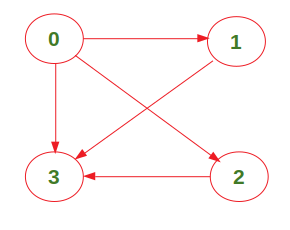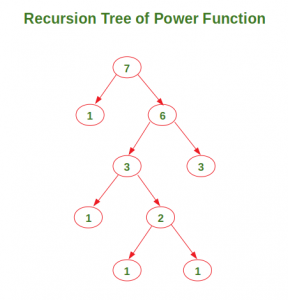# Number of Walks from source to destination

Given a graph and two vertices src and dest, count the total number of paths from src to dest where the length of the path is k (there should be exactly k edges between them). Note that the graph is represented as an adjacency matrix.

For example, consider the following graph:The number of paths from vertex 0 to vertex 3 with length 2 is 2 ({0->1->3} and {0->2->3}).

We have already discussed a O(V3 K) approach in count all possible walks from a source to a destination with exactly k edges. In this post, a O(V3 Log K) approach is discussed.

Approach: The idea is to compute a resultant matrix where. The total number of paths from source to destination of length k will then be simply. We use this technique to compute the exponentiation of the adjacency matrix of the given graph.

The recursion tree of power function used here for exponent = 7 looks like below:Below is the implementation of the above approach:

 `# Python3 code to count the number of paths  ` `# from the source to the destination of length ` `# k in the given graph ` ` `  `# Function to multiply two matrices ` `def` `multiply(mat1, mat2): ` `     `  `    ``result ``=` `[[``0` `for` `col ``in` `range``(``len``(mat2[``0``]))] \ ` `                    ``for` `row ``in` `range``(``len``(mat1))] ` `     `  `    ``for` `row ``in` `range``(``len``(mat1)): ` `         `  `        ``for` `col ``in` `range``(``len``(mat2[``0``])): ` `             `  `            ``for` `k ``in` `range``(``len``(mat2)): ` `                 `  `                ``result[row][col] ``+``=` `mat1[row][k] ``*` `mat2[k][col] ` `                 `  `    ``return` `result ` ` `  `# Function to calculate (matrix)^exp  ` `def` `power(matrix, exp): ` `     `  `    ``if` `exp ``=``=` `1``: ` `        ``return` `matrix ` `     `  `    ``else``:  ` `        ``tempMatrix ``=` `power(matrix, exp``/``/``2``) ` `        ``res ``=` `multiply(tempMatrix, tempMatrix) ` `         `  `        ``if` `exp ``%` `2` `=``=` `0``: ` `            ``return` `res ` `             `  `        ``else``: ` `            ``return` `multiply(matrix, res) ` `         `  `# Function to calculate total number of paths from  ` `# source to destination where length of path is k ` `def` `countWalks(graph, src, dest, k): ` `     `  `    ``resultantMatrix ``=` `power(graph, k) ` `    ``return` `resultantMatrix[src][dest] ` `     `  `# Driver code ` `graph ``=` `[[``0``, ``1``, ``1``, ``1``],  ` `         ``[``0``, ``0``, ``0``, ``1``],  ` `         ``[``0``, ``0``, ``0``, ``1``],  ` `         ``[``0``, ``0``, ``0``, ``0``]] ` `src, dest, k ``=` `0``, ``3``, ``2` `print``(``"Total number of walks:"``, countWalks(graph, src, dest, k)) `

Output:

```Total number of walks: 2
```

Time Complexity:Don’t stop now and take your learning to the next level. Learn all the important concepts of Data Structures and Algorithms with the help of the most trusted course: DSA Self Paced. Become industry ready at a student-friendly price.

My Personal Notes arrow_drop_upCheck out this Author's contributed articles.

If you like GeeksforGeeks and would like to contribute, you can also write an article using contribute.geeksforgeeks.org or mail your article to contribute@geeksforgeeks.org. See your article appearing on the GeeksforGeeks main page and help other Geeks.

Please Improve this article if you find anything incorrect by clicking on the "Improve Article" button below.

Article Tags :
Practice Tags :

3

Please write to us at contribute@geeksforgeeks.org to report any issue with the above content.